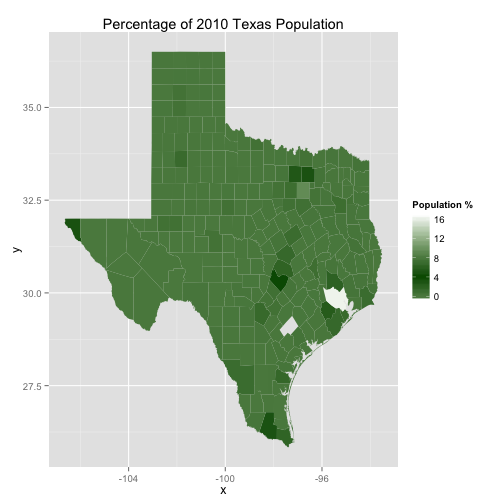First, download the Texas population by county here. Download it as a *.csv file and remove the line giving the results for the entire state.

``````texas.pop <- read.csv("2011_txpopest_county.csv", header = TRUE)
texas.pop\$census_2010_count.pcnt <- texas.pop\$census_2010_count/sum(texas.pop\$census_2010_count) *
100
``````

``````library(maptools)
texas.dist <- usa.dist[usa.dist\$NAME_1 == "Texas", ]
``````

Third, plot the map

``````library(ggplot2)
library(scales)
texas.fort <- fortify(texas.dist, region = "NAME_2")

ggplot() + geom_map(data = texas.pop, aes(map_id = county, fill = census_2010_count.pcnt),
map = texas.fort) + expand_limits(x = texas.fort\$long, y = texas.fort\$lat) +
labs(title = "Percentage of 2010 Texas Population") + scale_fill_gradient2(space = "Lab",
name = "Population %", limits = c(0, 17), midpoint = 4, high = "white",
mid = muted("darkgreen"), low = "white")
``````Published inR